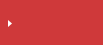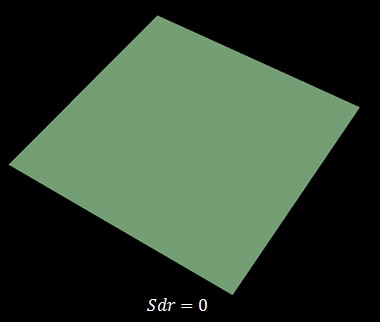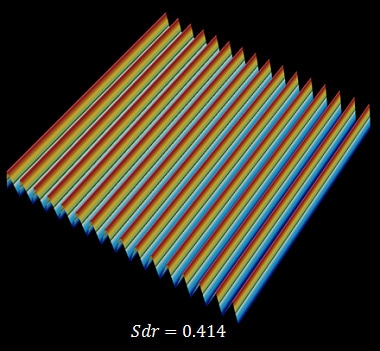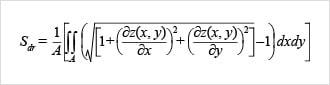>  What is area roughness?  >  Area Roughness Parameters

# Sdr (Developed Interfacial Area Ratio) | Area Roughness Parameters

## surface texture parameters in ISO 25178

• Height
• Spatial
• Hybrid
• Functional
• Functional volume
• FeatureSearch from the parameters list

## Sdr (Developed Interfacial Area Ratio)

This parameter is expressed as the percentage of the definition area's additional surface area contributed by the texture as compared to the planar definition area.

The Sdr of a completely level surface is 0.When a surface has any slope, its Sdr value becomes larger. The surface below is a plane with gradient components of 45 degrees and has an Sdr value of 0.414.## Search from the parameters list

Height Sa (arithmetical mean height) Sz (Maximum height) Sq (Root mean square height) Ssk (Skewness) Sku (Kurtosis) Sp (Maximum peak height) Sv (Maximum pit height) Sal (Auto-correlation length) / Str (Texture aspect ratio) Std* (Texture direction) Sdq (Root mean square gradient) Sdr (Developed interfacial area ratio) Smr(c) Areal material (bearing area) ratio Smc(mr) Inverse areal material ratio Sk(Core height) Spk (Reduced peak height) Svk (reduced dale height (reduced valley depth)) Smr (Peak material portion) Smr2 (Valley material portion) Sxp (Peak extreme height) Vvv (Dale void volume) Vvc (Core void volume) Vmp (Peak material volume) Vmc (Core material volume) Spd (Density of peaks) Spc (Arithmetic mean peak curvature) S10z / S5p / S5v / Sda(c) / Sha(c) / Sdv(c) / Shv(c)